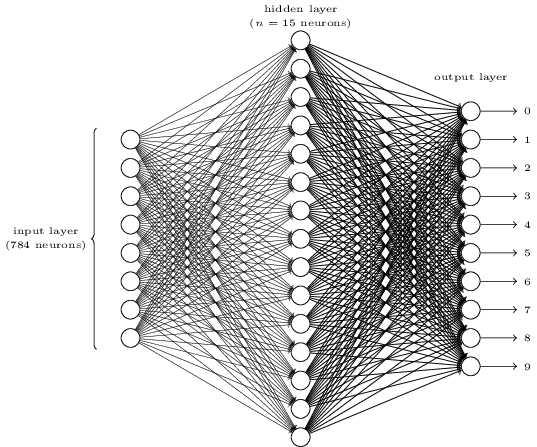# CS224N（1.24）Language Models and RNNs

\begin{eqnarray}P(x^{(t+1)}|x^{(t)},...,x^{(1)})\tag{1}\end{eqnarray}

$x^{(t+1)}$表示第$t+1$个位置（时刻）的词是$x$$x$可以是词典$V$中的任意一个词。

# CS224N（1.22）Dependency Parsing

Dependency Parsing是指对句子进行语法分析并画出句子成分的依赖关系，比如对于句子“She saw the video lecture”，首先可以分析出主语、谓语、宾语等句子成分；其次可以分析出依赖关系，比如saw依赖于She等。这就是句法分析。完成句法分析的算法被称为句法分析器parser，一个parser的性能可以用UAS和LAS，UAS就是parse出来的依赖关系对比正确依赖关系的正确率，LAS就是句子成分分析的正确率。

# Neural Networks and Deep Learning（七）番外篇·Pytorch MNIST教程

https://github.com/pytorch/examples/tree/master/mnist

# Neural Networks and Deep Learning（五）为什么深度神经网络难以训练Continue reading

# Neural Networks and Deep Learning（四）图解神经网络为什么能拟合任意函数

1. 并不是说神经网络可以精确计算任意一个函数$f(x)$，而是说当隐藏层神经元增加时，可以无限逼近$f(x)$，比如对于任何一个输入$x$，网络的输出$g(x)$和正确值$f(x)$的差小于某个阈值，$|g(x) - f(x)| < \epsilon$
2. 神经网络拟合的是连续函数，而不是那种不连续、离散、急剧变化的函数。Continue reading

# Neural Networks and Deep Learning（二）BP网络Continue reading